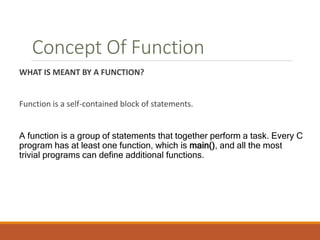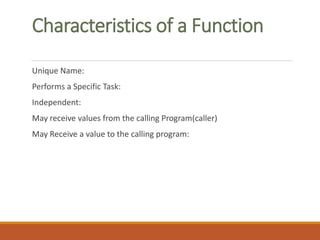Seu SlideShare está sendo baixado. ×

# CH.4FUNCTIONS IN C_FYBSC(CS).pptx

Anúncio
Anúncio
Anúncio
Anúncio
Anúncio
Anúncio
Anúncio
Anúncio
Anúncio
Anúncio
AnúncioCarregando em…3
×

1 de 31 Anúncio

# CH.4FUNCTIONS IN C_FYBSC(CS).pptx

The shared ppt contains the syllabus for the C Programming subject Ch no 4-Functions in C. It is helpful for FYBSC(CS) students

The shared ppt contains the syllabus for the C Programming subject Ch no 4-Functions in C. It is helpful for FYBSC(CS) students

Anúncio
Anúncio

### CH.4FUNCTIONS IN C_FYBSC(CS).pptx

1. 1. MIT ARTS,COMMERCE & SCIENCE COLLEGE,ALANDI(D),PUNE Department of Science & Computer Science F.Y.B.Sc(Computer Science) Problem Solving using Computers & C Programming” CH.4 FUNCTIONS
2. 2. Concept Of Function WHAT IS MEANT BY A FUNCTION? Function is a self-contained block of statements. A function is a group of statements that together perform a task. Every C program has at least one function, which is main(), and all the most trivial programs can define additional functions.
3. 3. Characteristics of a Function Unique Name: Performs a Specific Task: Independent: May receive values from the calling Program(caller) May Receive a value to the calling program:
4. 4. Types of Functions: Predefined or Standard Library Functions: For Ex: getch(),gets(),putch(),puts(),printf(),scanf() etc. User Defined Functions: The functions which we create for specific task is known as user defined functions.
5. 5. Advantages of Modular Design 1. Avoid redundancy 2. Reusability of code 3. Use of customized Library 4. Better Readability and Logical clarity 5. Easy maintenance and Debugging 6. Abstraction 7. Portability
6. 6. Standard Library Functions Examples: Islower(int c) Isspace() Isupper() Isdigit() Isalpha()
7. 7. User Defined Functions To define user defined functions, User have to follow three steps: Step 1: Function declaration or function prototype. Step 2: Function definition or function body Step 3: calling of function.
8. 8. Steps in User Defined Functions Func2() { Function body; } Void Func1(); Void Func2(); Main() { … Func1(); Func2(); … } Func1() { Function body; } Main Program Function definition
9. 9. Function Declarations or Function Prototype Function Declaration informs the compiler about the following 3 thing 1. Name of the function 2. Number and type of argument received by the function. 3.Type of value returned by the function.
10. 10. Function Declaration Syntax: Return_type function_name(datatype arg1,datatype arg2…..datatype argn);
11. 11. Function Declaration Syntax: A function definition in C programming consists of a function header and a function body. Here are all the parts of a function − return_type function_name( parameter list ) { body of the function } Where, Return Type − A function may return a value. The return type is the data type of the value the function returns. Some functions perform the desired operations without returning a value. In this case, the return type is the keyword void. Function Name − This is the actual name of the function. The function name and the parameter list together constitute the function signature. continue…
12. 12. Parameters − A parameter is like a placeholder. When a function is invoked, you pass a value to the parameter. This value is referred to as an actual parameter or argument. The parameter list refers to the type, order, and a number of the parameters of a function. Parameters are optional; that is, a function may contain no parameters. Function Body − The function body contains a collection of statements that define what the function does.
13. 13. Function Definition: Syntax: The general form of a function definition in C programming language is as follows − return_type function_name( parameter list ) { body of the function }
14. 14. Defining a Function: Example * /function returning the max between two numbers */ int max(int num1, int num2) { /* local variable declaration */ int result; if (num1 > num2) result = num1; else result = num2; return result; }
15. 15. Function Declarations A function declaration tells the compiler about a function name and how to call the function. The actual body of the function can be defined separately. A function declaration has the following parts − return_type function_name( parameter list ); For the above defined function max(), the function declaration is as follows − int max(int num1, int num2); Parameter names are not important in function declaration only their type is required, so the following is also a valid declaration − int max(int, int); Function declaration is required when you define a function in one source file and you call that function in another file. In such case, you should declare the function at the top of the file calling the function.
16. 16. C - Scope Rules A scope in any programming is a region of the program where a defined variable can have its existence and beyond that variable it cannot be accessed. There are three places where variables can be declared in C programming language − Inside a function or a block which is called local variables. Outside of all functions which is called global variables. In the definition of function parameters which are called formal parameters.
17. 17. Calling a Function While creating a C function, you give a definition of what the function has to do. To use a function, you will have to call that function to perform the defined task. When a program calls a function, the program control is transferred to the called function. A called function performs a defined task and when its return statement is executed or when its function-ending closing brace is reached, it returns the program control back to the main program. To call a function, you simply need to pass the required parameters along with the function name, and if the function returns a value, then you can store the returned value.
18. 18. PROGRAM TO FIND OUT MAXIMUM NUMBER AMOUNG TWO NUMBERS USING FUNCTION. Ex: 20 50 Max: 50 #include <stdio.h> void max(int num1, int num2); /* function declaration */ int main () { /* local variable definition */ int a = 100; int b = 200; int ret; ret = max(a, b); /* calling a function to get max value */ printf( "Max value is : %dn", ret ); } /* function returning the max between two numbers */ int max(int num1, int num2) { int result; /* local variable declaration */ if (num1 > num2) result = num1; else result = num2; return result; }
19. 19. Local Variables Variables that are declared inside a function or block are called local variables. They can be used only by statements that are inside that function or block of code. Local variables are not known to functions outside their own.
20. 20. Example: #include <stdio.h> int main () { /* local variable declaration */ int a, b; int c; /* actual initialization */ a = 10; b = 20; c = a + b; printf ("value of a = %d, b = %d and c = %dn", a, b, c); return 0; }
21. 21. Global Variables Global variables are defined outside a function, usually on top of the program. Global variables hold their values throughout the lifetime of your program and they can be accessed inside any of the functions defined for the program.
22. 22. Global Variables:Example #include <stdio.h> /* global variable declaration */ int g; int main () { /* local variable declaration */ int a, b; /* actual initialization */ a = 10; b = 20; g = a + b; printf ("value of a = %d, b = %d and g = %dn", a, b, g); }
23. 23. Function Arguments If a function is to use arguments, it must declare variables that accept the values of the arguments. These variables are called the formal parameters of the function. Formal parameters behave like other local variables inside the function and are created upon entry into the function and destroyed upon exit.
24. 24. While calling a function, there are two ways in which arguments can be passed to a function− By default, C uses call by value to pass arguments. In general, it means the code within a function cannot alter the arguments used to call the function. 1. Call By Value 2. Call By Reference
25. 25. call by value The call by value method of passing arguments to a function copies the actual value of an argument into the formal parameter of the function. In this case, changes made to the parameter inside the function have no effect on the argument.
26. 26. By default, C programming uses call by value to pass arguments. function definition to swap the values void swap(int x, int y) { int temp; temp = x; x = y; y = temp; printf(“x:%d y:%d”x,y); } Void main() { printf(“E Swap(x,y); }
27. 27. #include <stdio.h> /* function declaration */ void swap(int x, int y); int main () { /* local variable definition */ int a = 100; int b = 200; swap(a, b); printf("After swap, value of a : %dn", a ); printf("After swap, value of b : %dn", b } void swap(int x, int y) { int temp; temp = x; x = y; y = temp; }
28. 28. call by reference The call by reference method of passing arguments to a function copies the address of an argument into the formal parameter. Inside the function, the address is used to access the actual argument used in the call. It means the changes made to the parameter affect the passed argument.
29. 29. /* function definition to swap the values */ void swap(int *x, int *y) { int temp; temp = *x; /* save the value at address x */ *x = *y; /* put y into x */ *y = temp; /* put temp into y */ }
30. 30. Call by reference #include <stdio.h> int main () { /* local variable definition */ int a = 100; int b = 200; printf("Before swap, value of a : %dn", a ); printf("Before swap, value of b : %dn", b ); /* calling a function to swap the values */ swap(&a, &b); printf("After swap, value of a : %dn", a ); printf("After swap, value of b : %dn", b ); return 0; } void swap(int *x, int *y) { int temp; temp = *x;
31. 31. THANK YOU.